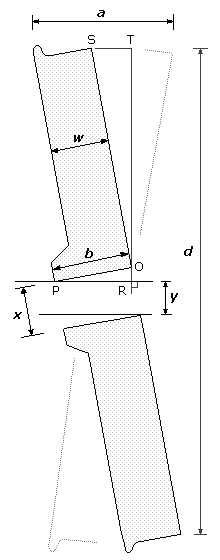# Assessment of the fit of a bore on a shaft

###### by Russ Elliott

This page gives a method of assessing the difference between the size of a parallel bore,  x, and the size of a shaft,  y, where the exact bore size is not known and where it is not a tight or interference fit on the shaft.

In the example of the wheel shown, the wheel is first tipped to one side so that the extremities of its bore touch the surface of the shaft, and then tipped to the other side, and a measurement is made, at the diameter of the wheel, of the distance  a, the extent of the tip over the outer surfaces of the wheel.

Triangles OPR and OST are similar, therefore the ratio of ST to OT is equal to the ratio of OR to PR, i.e. ST ∕ OT = OR ∕ PR

• If the angle of the tipped wheel is very small:
ST = (aw) ∕ 2

• If OR is very small compared to PR:
OR =  xy ; and b = PR

• If OR is very small compared to OT:
OT = ( dy) ∕ 2
where  d is the diameter of the wheel

Re-expressing the congruency,  xy, the difference between the bore and shaft sizes, is:

xy =  baw) ∕ ( dy)